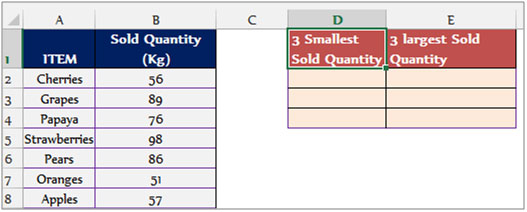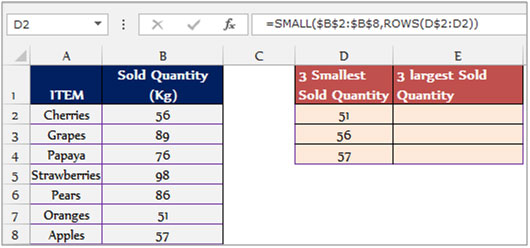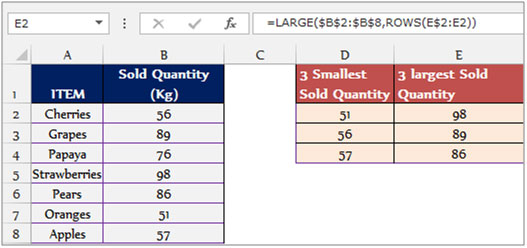# Sorting Numbers in Ascending or Descending Order in Microsoft Excel

To sort the numbers in ascending or descending order, we use the formulas “LARGE”, “SMALL” and “ROW” in Microsoft Excel.

To sort in ascending order use the “SMALL” function along with the “ROW” function. And to sort in descending order use the “LARGE” function along with the “ROW” function.

To understand how you can sort numbers in ascending or descending order, let’s take an example.

Example 1: We have a list of fruitsalong with the sold quantity;Column “A” contains the names of the fruits and column “B” the sold quantity.

In column D we need to return the 3 Smallest sold quantity and in column E we need to return the 3 largest sold quantity.How arrange the data in ascending order through formula?

To sort the data in ascending order through formula follow the below mentioned steps:-

• Select the cell D2 and write the formula
• =SMALL(\$B\$2:\$B\$8,ROWS(D\$2:D2))
• Press Enter on the keyboard.
• The function will return the first smallest sold quantity
• To return the 2nd and 3rd smallest quantity, copy the same formula by pressing the key “CTRL+C” and select the range D3:D4 and paste by pressing the key “CTRL+V” on the keyboard.How arrange the data in descending order through formula?

To sort the data in descending order through formula follow the below mentioned steps:-

• Select the cell E2 and write the formula
• =LARGE(\$B\$2:\$B\$8,ROWS(E\$2:E2))
• Press enter on the keyboard.
• The function will return the first largest sold quantity
• To return the 2nd and 3rd largest quantity, copy the same formula by pressing the key “CTRL+C” and select the range E3:E4 and paste by pressing the key “CTRL+V” on the keyboard.## Users are saying about us...

1.I Have stress Strain data (some 5000 rows in excel) & want to sort it in ascending order. Both columns ( for stress - Strain) are inter related. Ex. Val in 8th row strain column corresponds to value in 8th row stress column.

IMPORTANT: -- I want to ignore rows in between for which values are going in descending order & jump to next row with ascending order to form two new columns with ascending order.

Is it possible with excel formulation?

2.Please help - I am trying to do the same thing as this EXCEPT for your exmple I would be trying to find out which item (text) is has the highest value next to it.

I cannot do it manually by selecting my data and sorting it because I need it to do this automatically.

Anyone can help?

Thanks,
Yasmine

•Hi Yasmine,

Kindly post you query @ http://www.excelforum.com . You will get the appropriate reply immedialtely.

Regards
Nisha

3.I would like to get the PO numbers(C) for a given DrawID(A) & Material(B) in column F,G,H.

A B C E F G
Draw ID Material PO No
LMV/123456 PICS163011436 2345678
LMV/000000 PICS163011436 2345679
LMV/123456 PICS163000000 2345680
LMV/123456 PICS163011436 2345681

4.How to get name of item with Rank or Large formula...

5.How will get the Item name with Rank or Large Formula?

6.I have 1200 numbers that I need to formulate in either descending or ascending order.

•Hi Noni Campbell,

To sort the numbers in descending or ascending order, please use the shortcut ALT+D+S, which will open up a sorting window. This window will enable you to sort the numbers as per your requirement from smallest to largest or largest to smallest.

Happy Learning,
•